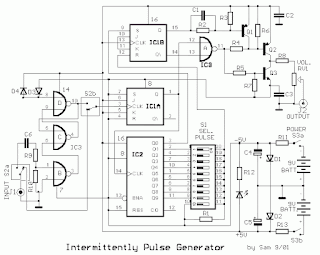## Sunday, November 17, 2013

Browse » home» » » » » Alternating Square Pulse Generator

### Alternating Square Pulse Generator

The generator circuit was designed to produce alternating square pulses with vibrations in different parts of the circuit.

4027 – a dual JK flip flop that has independent clock, set, and reset inputs for each flip flop used in toggle, register, and control functions due to its features such as capability of driving two low power TTL loads, logic edge clocked flip flop design, logic swing independent of fanout, toggle rate of 3 MHz at 5 Vdc, supply voltage range of 3 V to 6 V, protection of diodes on all inputs, noise immunity, and quiescent current of 2 nA at 5 Vdc. 4001 – a quad 2-input NOR gate integrated circuit, generally characterized by small fluctuation in voltage supply, very high impedance, outputs that can sink and source, one output can drive up to 50 inputs, high speed gate propagation time, high frequency, and low power consumption.

Alternating Square Pulse Generator Circuit DiagramBC550 – an NPN general purpose transistor with low current and low voltage used for low noise stages in audio frequency equipment

The generator functions without distortion when it reaches 100 KHz. The square pulses are produced when the selector switch S1 is turned ON, which matches the output of Q3 at pin 7. The 5V pulse line is applied to J1 input, the signal is fed to T-flip flop IC1A through S2 which creates pulses indicated at half of the duration time where pin 2 handles the division of pulse frequency. The pulses are applied to IC2 pin 14 and IC3A input. IC2 acts as decimal counter with decode outputs where each entry produces HIGH on one of the outputs. From the initial pulse entry, the output of Q1 becomes HIGH while others are LOW. The second pulse entry causes HIGH output on Q2, and third entry applies on Q3. The same operation occurs for the succeeding pulse line entry, since the counter checks the number of pulses that passes the generator output.

The generator output stage is considered on levels where the driving stage of Q2 creates positive output voltage and the saturation of Q 3 in the cutoff region. Through the potentiometer, the signal is applied in the output of J2. Using the gates of IC3B-C-D, the sine wave or triangular wave can be changed to square pulses in the circuit input. The conversion is directly made from the command of S2. The switching can also be done by DIP switch S2. A suitable power supply or two NiCd batteries can maintain the circuit with the stabilization of voltage achieved by two Zener diodes.

R1-10= 10Kohm
R2= 47Kohm
R3= 22Kohm
R4-5= 18Kohm
R6-7= 4.7Kohm
R8= 1.2Kohm
R10= 100Kohm
R11-13= 470 ohm
R12= 1.5Kohm RV1= 1.2Kohm linear pot.
C1= 15pF ceramic
C2-3= 10nF 63V MKT
C4-5= 470uF 16V
C6= 1uF 63V MKT
D1-2= 5.1V 0.5W zener
D3-4= 1N4148
Q1-2= BC560C
Q3= BC550C IC1= 4027
IC2= 4027
IC3= 4001B
S1= switch DIL 10S
S2-3= 2X2 mini switch
LD1= LED
BATT= 9V Battery NiCd

The generation of square pulses can bring about plenty of usages by adjusting the input in digital circuits and controlling the frequencies of amplifiers, loudspeakers, rooms of hearings, and others. One popular application is in the camera flash temporal profile where square pulses act as a heating source in the photoflash technique. The amount of light emitted by an electronic flash is controlled by the shunted output of a pulse generator known as tailbiters where longer pulses are made with the biting off the tail of the impulse.